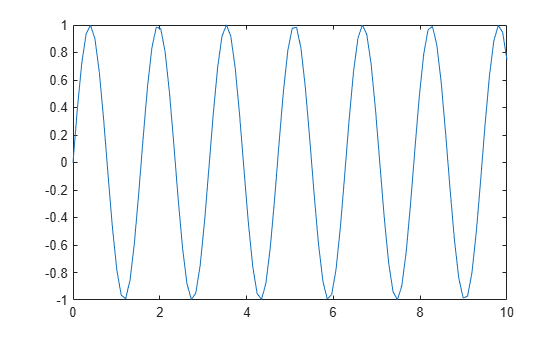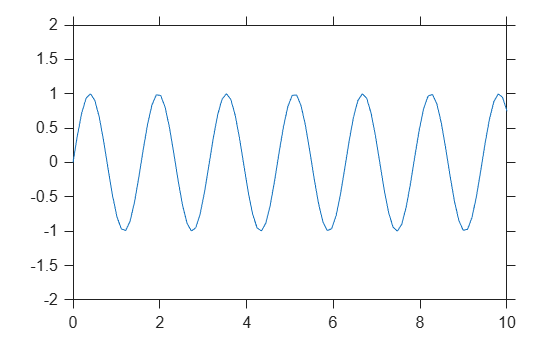# gca

Current axes or chart

## Syntax

``ax = gca``

## Description

example

````ax = gca` returns the current axes (or standalone visualization) in the current figure. Use `ax` to get and set properties of the current axes. If there are no axes or charts in the current figure, then `gca` creates a Cartesian axes object.```

## Examples

collapse all

Plot a sine wave.

```x = linspace(0,10); y = sin(4*x); plot(x,y)```Set the font size, tick direction, tick length, and y-axis limits for the current axes. Use `gca` to refer to the current axes.

```ax = gca; % current axes ax.FontSize = 12; ax.TickDir = 'out'; ax.TickLength = [0.02 0.02]; ax.YLim = [-2 2];```## Output Arguments

collapse all

Current axes, returned as an `Axes` object, a `PolarAxes` object, a `GeographicAxes` object, or a standalone visualization such as a `heatmap`.

collapse all

### Current Axes

The current axes is the default target object for many graphics commands, such as `plot`, `title`, and `xlim`. The following types of objects can become the current axes. Typically, it is the last one of these objects that is created, clicked on, or plotted into.

• An `Axes` object.

• A `PolarAxes` object.

• A `GeographicAxes` object.

• A standalone visualization, which is a chart designed for a special purpose that works independently from other charts. For example, a `heatmap` is a standalone visualization for observing the interaction between two variables in tabular data.

The `CurrentAxes` property of a figure stores its current axes.

## Tips

• User interaction can change the current axes or chart. It is better to assign the axes or chart to a variable when you create it instead of relying on `gca`.

• Changing the current figure also changes the current axes or chart.

• Set axes properties after plotting since some plotting functions reset axes properties.

• To access the current axes or chart without forcing the creation of Cartesian axes, use dot notation to query the figure `CurrentAxes` property. MATLAB® returns an empty array if there is no current axes.

```fig = gcf; ax = fig.CurrentAxes;```

### Properties

Introduced before R2006a